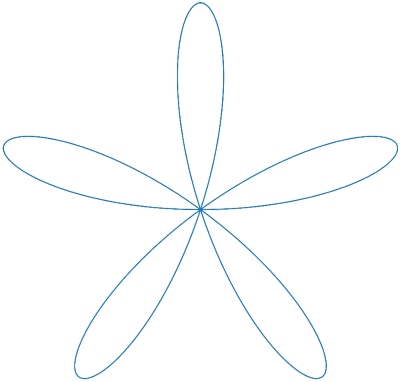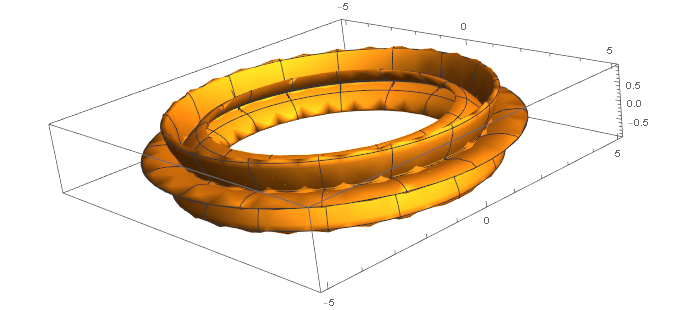# Volume of a rose-shaped torusNow spin that rose around a vertical line a distance R from the center of the rose. This makes a torus (doughnut) shape whose cross sections look like the rose above. You could think of having a cutout shaped like the rose above and extruding Play-Doh through it, then joining the ends in a loop.In case you’re curious, the image above was created with the following Mathematica command:

`      RevolutionPlot3D[{4 + Cos[5 t] Cos[t], Cos[5 t] Sin[t]}, {t, 0, 2 Pi}]`

What would the volume of resulting solid be?

This sounds like a horrendous calculus homework problem, but it’s actually quite easy. A theorem by Pappus of Alexandria (c. 300 AD) says that the volume is equal to the area times the circumference of the circle traversed by the centroid.

The area of a rose of the form r = cos(kθ) is simply π/2 if k is even and π/4 if k is odd. (Recall from the previous post that the number of petals is 2k if k is even and k if k is odd.)

The location of the centroid is easy: it’s at the origin by symmetry. If we rotate the rose around a vertical line x = R then the centroid travels a distance 2πR.

So putting all the pieces together, the volume is π²R if k is even and half that if k is odd. (We assume R > 1 so that the figure doesn’t intersect itself and some volume get counted twice.)

We can also find the surface area easily by another theorem of Pappus. The surface area is just the arc length of the rose times the circumference of the circle traced out by the centroid. The previous post showed how to find the arc length, so just multiply that by 2πR.